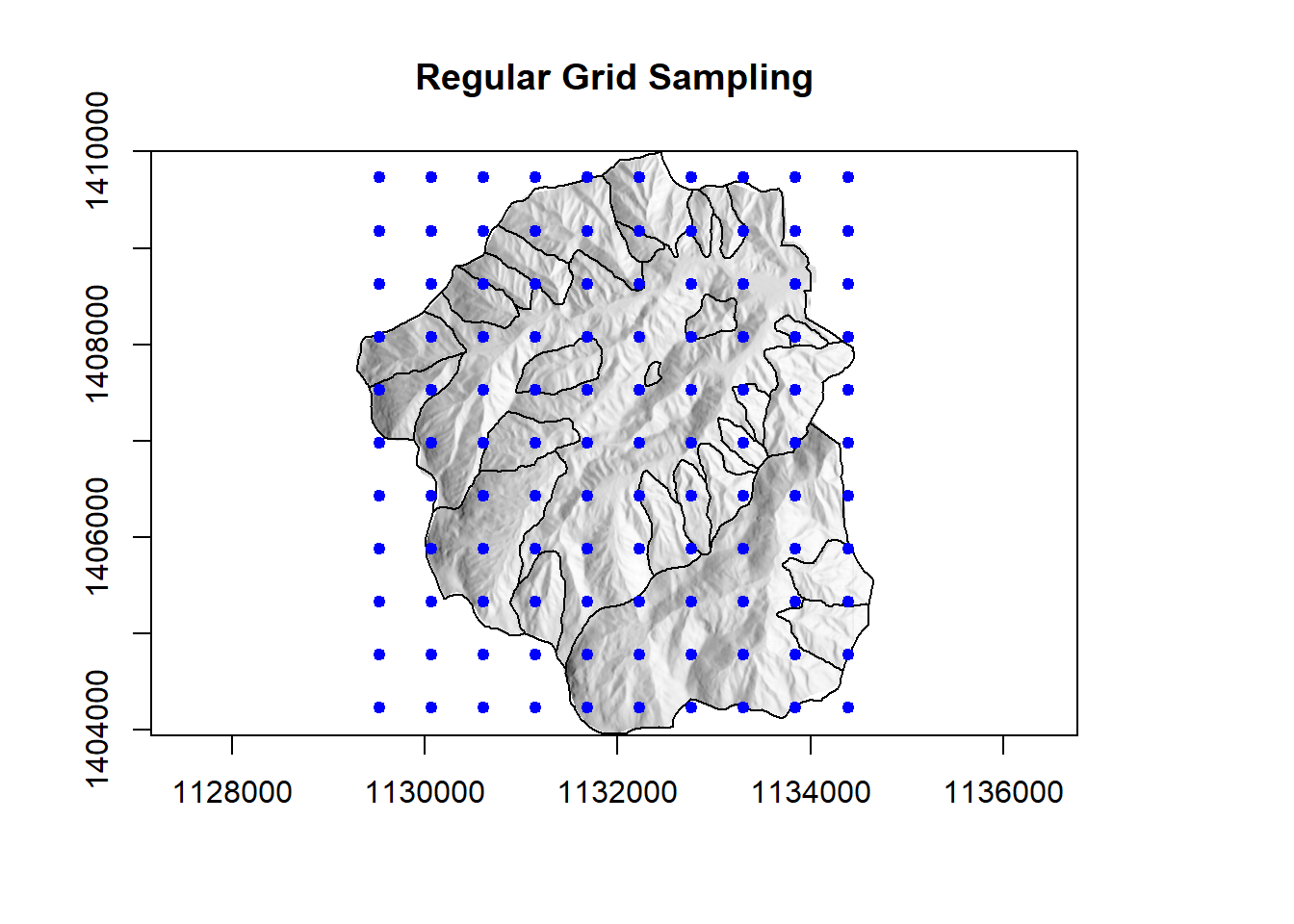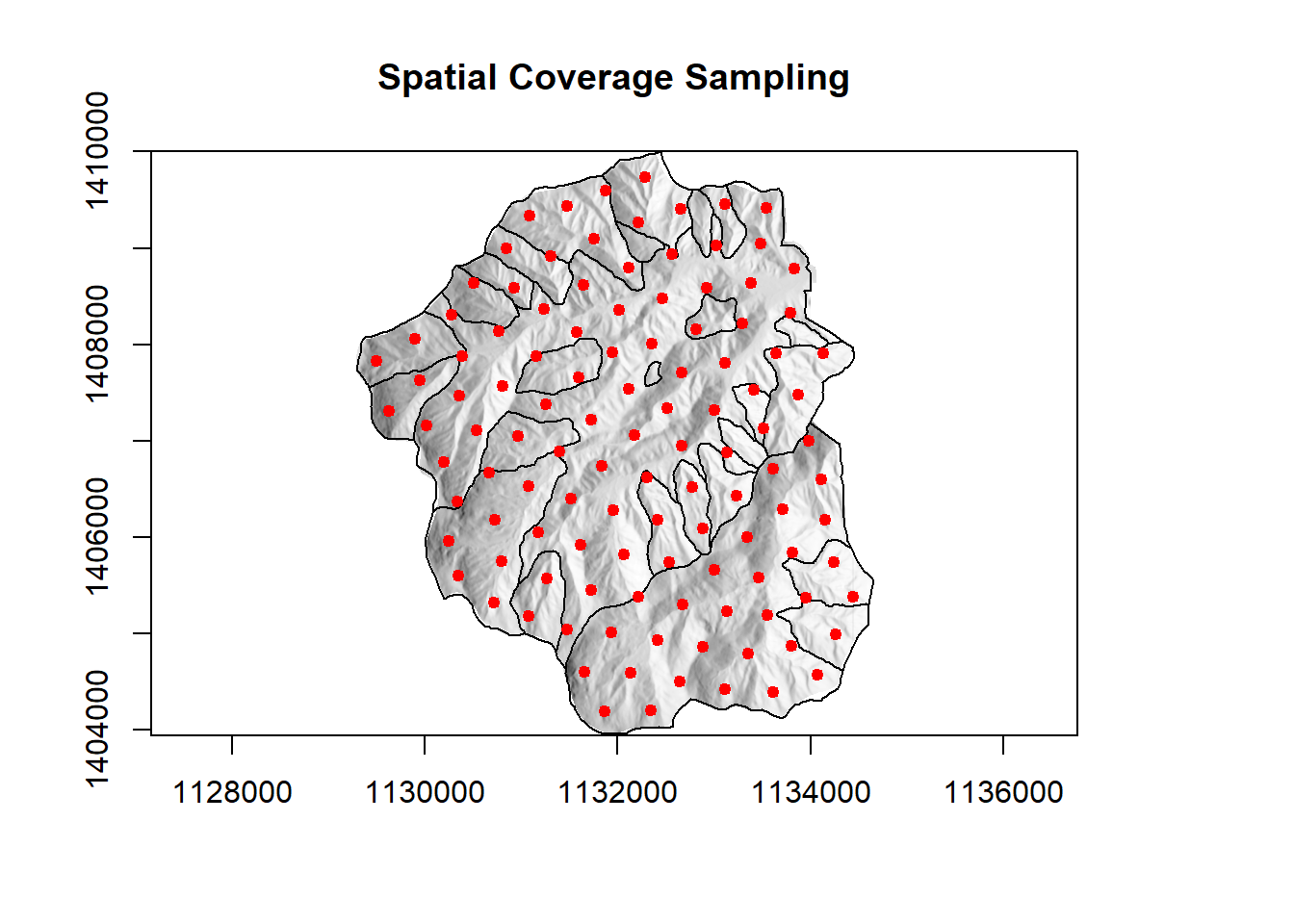# Raster Mastery Issue 3Issue 3 Having trouble viewing this email? View it as a Web page.# Sampling Techniques: When a Grid Can Be So Much More

Sampling is a key component to digital soil mapping projects. It’s also a topic that is often discussed amongst practitioners…for hours upon hours actually. Numerous tools are at our disposal when designing a sampling scheme, but we often don’t consider grid sampling as a first-line strategy for sampling design. In this issue of Raster Mastery, we ask…should I grid sample? If so, is there an optimal way to generate grid samples? What if I want to incorporate covariate data? We have so many options to consider! Read on as we explore the options in R (insert super enthusiastic R emoji here).

First, let’s set up our working environment and bring in some data.

``````# load libraries
library(raster);library(rgdal);library(clhs);library(spcosa); library(LICORS); library(parallel)

dem <- raster("C:/rwork/coweeta/chldem10m.tif")
# create slope and aspect rasters
slp <- terrain(dem, opt="slope")
aspt <- terrain(dem, opt="aspect")
hill <- hillShade(slp, aspt, angle = 40, direction = 270)``````

## Regular Grid Sampling

Traditional grid sampling can be useful for a detailed investigation. However, sometimes getting an exact number of points you want to fall within a regular grid can be very difficult. The following creates a regular grid of 115 points over the DEM for our project location.

``````# create a regular saple of points
grd_pts <- sampleRegular(dem, size = 115, sp=T)``````Notice that, although we wanted to sample 115 points, many are outside of our project area boundary. Why though? The algorithm uses the bounding box, rather than the project area boundary, as the spatial constraint. That won’t do. We need a smarter grid! We can take a different approach by utilizing the spatial coverage sampling scheme in the spcosa R package.

## Spatial Coverage Sampling

This process uses k-means clustering to generate points based on the geometry of the raster data. Smarter grid for sure.

``````# convert raster to data.frame
grd <- as.data.frame(dem, xy=T)
grd <- na.omit(grd) #remove NAs
gridded(grd) <- ~ x * y # convert to grid format
# function
sc_grd <- stratify(grd, nStrata = 115, nTry=10)

# pull out sample points and save to shp
samp_pattern <- spsample(sc_grd)
samp_pts <- as(samp_pattern, "data.frame")
coords<- samp_pts[c("x","y")]
prj <- dem@crs
samp_pts <- SpatialPointsDataFrame(coords = coords, data = samp_pts, proj4string = prj)``````The resulting grid is a set of points that are equally distributed throughout the project area, and each in the center of a k-means cluster. Now that’s better! What if we kicked it up a notch? Let’s sample based on the environmental covariates developed to represent important soil forming factors. One popular technique is conditioned Latin hypercube sampling(cLHS), another technique is feature space coverage sampling (FSCS).

## Feature Space Coverage Sampling

The spatial coverage sampling technique uses k-means to find the shortest distance between sample locations and the XY coordinates of a raster. FSCS builds off this technique by using k-means to find the optimal coverage spanned by the environmental covariates.

First, let’s set up the covariate data.

``````# set directory to covariates
setwd("C:/rwork/coweeta/cov/cov")

# read in raster layers names and create list
rlist=list.files(getwd(), pattern=".tif\$", full.names = FALSE)

# create raster stack
rstk <- stack(rlist)

# create a grid of points (n=gsize) to represent the raster stack
# - this can be done many ways sampleRegular is one method
gsize <- 10000
samp_reg <- sampleRegular(rstk, size = gsize, sp=T)

samp_regdf <- na.omit(as.data.frame(samp_reg))``````

Stack ’em up! To run FSCS, we need to convert the raster stack to a data frame in R. For spatially large rasters and for raster stacks with numerous covariates, the conversion is a time-consuming process. That might be welcome if you enjoy the art of slow coffee, but it’s not great for productivity. We recommend you slam that coffee and create a regular grid of points to represent the covariate data. You can set the size of the grid in the gsize parameter.

Next let’s run the FSCS algorithm… in parallel to be even more productive.

``````# parallel implementation of FSCS
nw <- detectCores(logical = F)-1
cl <- makeCluster(nw)
clusterSetRNGStream(cl, iseed=1234)
set.seed(88)
# Parallelize over the "nstart" argument
nstart <- 100
# Create vector of length "nw" where sum(nstartv) == nstart
nstartv <- rep(ceiling(nstart / nw), nw)
results <- clusterApply(cl, nstartv,
function(n,x) LICORS::kmeanspp(x, 115, nstart=n, iter.max=1000),
samp_regdf[,1:4])
# Pick the best result
i <- sapply(results, function(result) result\$tot.withinss)
result <- results[[which.min(i)]]
stopCluster(cl)``````

The key parameters (underlined below) for the kmeanspp function in the parallel implementation (code block above) of the FSCS algorithm are:
nstart 100 # number of random starts
sampNo 115 # number of clusters whose centroids represent sampling locations
iter.max 1000 # number of iterations
samp_regdf[,1:4] columns in our dataframe representing the covariates

Finally, let’s pull out the FSCS points.

``````# pull out the points
fscs <- as.data.frame(result\$inicial.centers)
cov <- names(fscs)
fscs.pts <- merge(samp_regdf, fscs, by = cov)

# projection information
prj <- rstk@crs
coords <- fscs.pts[c("x","y")]
# convert to sp
fscs.pts <- SpatialPointsDataFrame(coords = coords, data = fscs.pts, proj4string = prj)

# save pts to shpfile
writeOGR(fscs.pts, dsn = ".", layer="fscs135.pts", driver = "ESRI Shapefile", overwrite_layer = T)``````